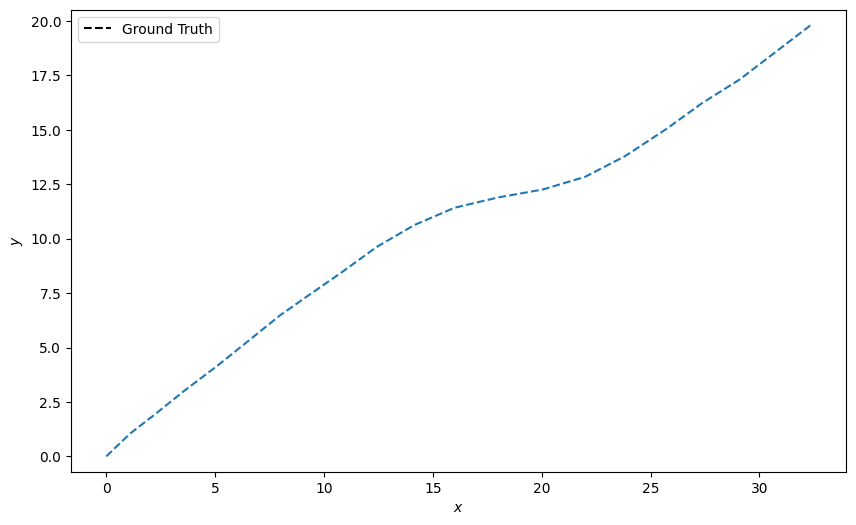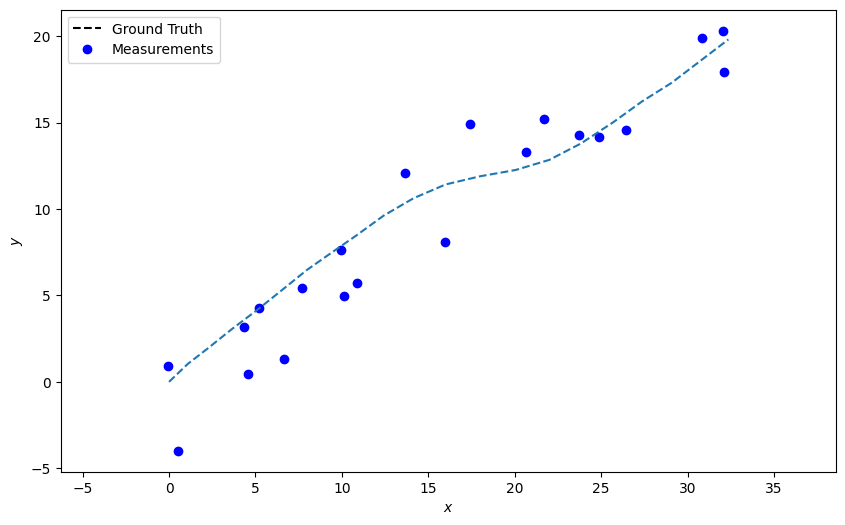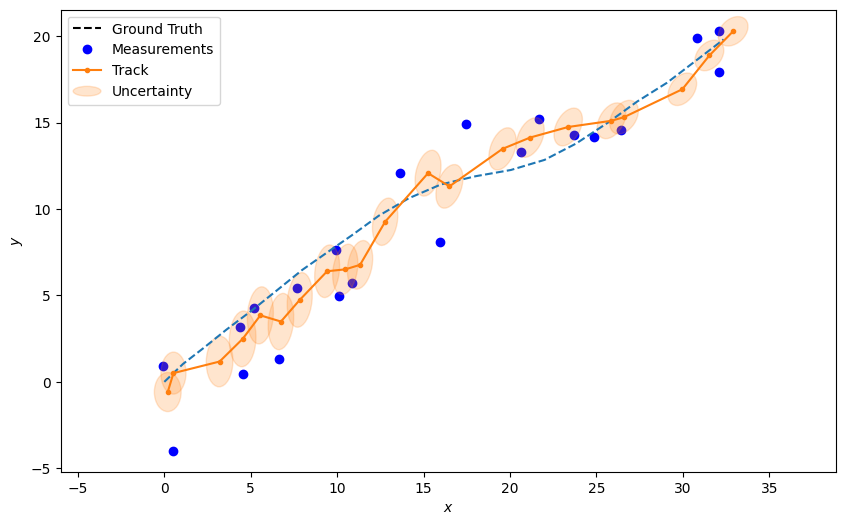# 2 - Non-linear models: extended Kalman filter¶

As well as introducing various aspects of the Stone Soup framework, the previous tutorial detailed the use of a Kalman filter. A significant problem in using the Kalman filter is that it requires transition and sensor models to be linear-Gaussian. In practice, many models are not like this and so alternatives are required. We examine the most commonly-used of such alternatives, the extended Kalman filter 1 (EKF), in this tutorial.

## Background¶

Recall that the Kalman filter makes the following linear assumptions of the predicted state and predicted measurement:

$\begin{split}\mathbf{x}_{k|k-1} &= F_{k} \mathbf{x}_{k-1} + B_{k}\mathbf{u}_{k}\\ \mathbf{z}_{k|k-1} &= H_{k} \mathbf{x}_{k|k-1}\end{split}$

The EKF gets round the fact that $$f(\cdot)$$ and $$h(\cdot)$$ are not of this form by making linear approximations about $$\mathbf{x}_{k−1} = \boldsymbol{\mu}_{k−1}$$ or $$\mathbf{x}_{k|k−1} = \boldsymbol{\mu}_{k|k−1}$$. It does this by way of a Taylor expansion,

$g(\mathbf{x})\rvert_{\mathbf{x}=\boldsymbol{\mu}} \approx \sum\limits_{|\alpha| \ge 0} \frac{(\mathbf{x} - \boldsymbol{\mu})^{\alpha}}{\alpha !} (\mathcal{D}^{\alpha} g)(\boldsymbol{\mu})$

This is usually truncated after the first term, meaning that either $$\tilde{F}(\mathbf{x}_{k-1}) \approx J(f)\rvert_{\mathbf{x}=\boldsymbol{\mu}_{k-1}}$$ or $$\tilde{H}(\mathbf{x}_{k|k-1}) \approx J(h)\rvert_{\mathbf{x}=\boldsymbol{\mu}_{k|k-1}}$$ or both, where $$J(\cdot)$$ is the Jacobian matrix. The calculation of the covariances, including the innovation covariance, then proceeds in exactly the same way as in the Kalman filter using these approximations,

$\begin{split}\mathbf{x}_{k|k-1} &= f_{k}(\mathbf{x}_{k-1}) \\ P_{k|k-1} &= \tilde{F}_{k}P_{k-1}\tilde{F}_{k}^T + Q_{k}\\ \mathbf{x}_{k} &= \mathbf{x}_{k|k-1} + \tilde{K}_k(\mathbf{z}_k - h_k(\mathbf{x}_{k|k-1}))\\ P_{k} &= P_{k|k-1} - \tilde{K}_k \tilde{H}_{k} P_{k|k-1}\\\end{split}$

where,

$\begin{split}\tilde{K}_k &= P_{k|k-1} \tilde{H}_{k}^T \tilde{S}_k^{-1}\\ \tilde{S}_k &= \tilde{H}_{k} P_{k|k-1} \tilde{H}_{k}^T + R_{k}\end{split}$

(we omit the control term in this analysis but it also can be incorporated as a non-linear approximation of the same form.)

Stone Soup implements the EKF for non-linear functions using a finite difference method to find $$J(\cdot)$$ in the appropriate places. We’ll now see this in action.

## Nearly-constant velocity example¶

We’re going to use the same target model as previously, but this time we use a non-linear sensor model.

import numpy as np
from datetime import datetime, timedelta
start_time = datetime.now()

np.random.seed(1991)


### Create a target¶

As in the previous tutorial, the target moves with near constant velocity NE from 0,0.

from stonesoup.models.transition.linear import CombinedLinearGaussianTransitionModel, \
ConstantVelocity
from stonesoup.types.groundtruth import GroundTruthPath, GroundTruthState
transition_model = CombinedLinearGaussianTransitionModel([ConstantVelocity(0.05),
ConstantVelocity(0.05)])
truth = GroundTruthPath([GroundTruthState([0, 1, 0, 1], timestamp=start_time)])

for k in range(1, 21):
truth.append(GroundTruthState(
transition_model.function(truth[k-1], noise=True, time_interval=timedelta(seconds=1)),
timestamp=start_time+timedelta(seconds=k)))


Plot this

from stonesoup.plotter import Plotter
plotter = Plotter()
plotter.plot_ground_truths(truth, [0, 2])### A bearing-range sensor¶

We’re going to simulate a 2d bearing-range sensor. An example of this is available in Stone Soup via the CartesianToBearingRange class. As the name suggests, this takes the Cartesian state input and returns a relative bearing and range to target. Specifically,

$\begin{split}\begin{bmatrix} \theta\\ r\\ \end{bmatrix} = \begin{bmatrix} \arctan\left(\frac{y-y_p}{x-x_p}\right)\\ \sqrt{(x-x_p)^2 + (y-y_p)^2} \end{bmatrix}\end{split}$

where $$x_p,y_p$$ is the 2d Cartesian position of the sensor and $$x,y$$ that of the target. Note also that the arctan function has to resolve the quadrant ambiguity and so is implemented as the arctan2$$(y,x)$$ function in Python.

from stonesoup.models.measurement.nonlinear import CartesianToBearingRange
sensor_x = 50  # Placing the sensor off-centre
sensor_y = 0

measurement_model = CartesianToBearingRange(
ndim_state=4,
mapping=(0, 2),
noise_covar=np.diag([np.radians(0.2), 1]),  # Covariance matrix. 0.2 degree variance in
# bearing and 1 metre in range
translation_offset=np.array([[sensor_x], [sensor_y]])  # Offset measurements to location of
# sensor in cartesian.
)


We create a set of detections using this sensor model.

from stonesoup.types.detection import Detection

measurements = []
for state in truth:
measurement = measurement_model.function(state, noise=True)
measurements.append(Detection(measurement, timestamp=state.timestamp,
measurement_model=measurement_model))


Plot the measurements. Where the model is nonlinear the plotting function uses the inverse function to get coordinates.

plotter.plot_measurements(measurements, [0, 2])
plotter.fig### Set up the extended Kalman filter elements¶

Analogously to the Kalman filter, we now create the predictor and updater. As is our custom the predictor takes a transition model and the updater a measurement model. Note that if either of these models are linear then the extended predictor/updater defaults to its Kalman equivalent. In fact the extended Kalman filter classes inherit nearly all of their functionality from the Kalman classes. The only difference being that instead of returning a matrix, in the extended version the matrix() function returns the Jacobian.

from stonesoup.predictor.kalman import ExtendedKalmanPredictor
predictor = ExtendedKalmanPredictor(transition_model)

from stonesoup.updater.kalman import ExtendedKalmanUpdater
updater = ExtendedKalmanUpdater(measurement_model)


### Run the extended Kalman filter¶

First, we’ll create a prior state.

from stonesoup.types.state import GaussianState
prior = GaussianState([, , , ], np.diag([1.5, 0.5, 1.5, 0.5]), timestamp=start_time)


Next iterate over hypotheses and place in a track.

from stonesoup.types.hypothesis import SingleHypothesis
from stonesoup.types.track import Track

track = Track()
for measurement in measurements:
prediction = predictor.predict(prior, timestamp=measurement.timestamp)
hypothesis = SingleHypothesis(prediction, measurement)  # Group a prediction and measurement
post = updater.update(hypothesis)
track.append(post)
prior = track[-1]


Plot the resulting track complete with error ellipses at each estimate.

plotter.plot_tracks(track, [0, 2], uncertainty=True)
plotter.fig## Key points¶

1. Non-linear models can be incorporated into the tracking scheme through the use of the appropriate combination of Predictor/TransitionModel or Updater/MeasurementModel

2. Because Stone Soup uses inheritance, the amount of engineering required to achieve this is minimal and the interface is the same. A user can apply the EKF components in exactly the same way as the KF.

The first order approximations used by the EKF provide a simple way to handle non-linear tracking problems. However, in highly non-linear systems these simplifications can lead to large errors in both the posterior state mean and covariance. In instances where we have noisy transition, or perhaps unreliable measurement, this could lead to a sub-optimal performance or even divergence of the filter. In the next tutorial, we see how the unscented Kalman filter can begin to addresses these issues.